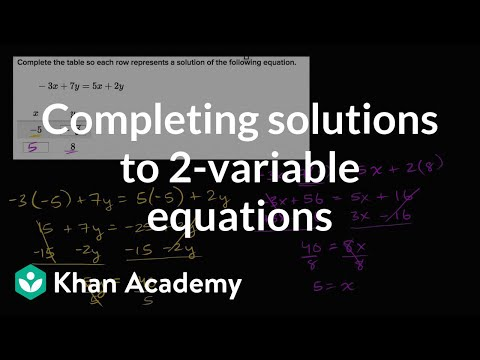Video

# Systems of equations with elimination: 3t + 4g = 6 and -6t + g = 6 (Full video)

Description: Sal demonstrates how to solve the system of equations 3t + 4g = 6 and -6t + g = 6 using the elimination method. But if I were to scale the 3 up, if I were to multiply it by 2 and it becomes 6t, and then I were to add these two things, then they would cancel out. So let me multiply this equation by 2. So I'm going to multiply 2 times 3t plus 4g is equal to 2 times 6.

### Other videos you might be interested in### Solving Systems of Equations by Substitution (Full video)

#### Student Achievement Partners